# 数据结构与算法： 最短路径

## Shortest Path

Posted by R1NG on February 20, 2022 Viewed Times

# 最短路径

## 1. 使用广度优先算法查找最短路径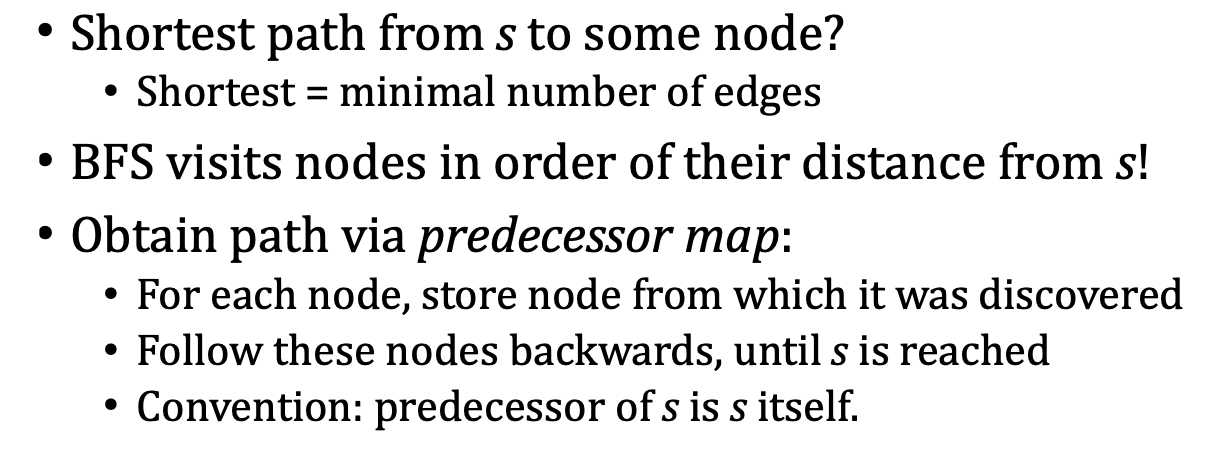1. ShortestPaths(s) 接受图的某个 没有父节点 的起始节点 $s$ 作为输入, 从 $s$ 开始 完整遍历整个图并将遍历到的每个节点的父节点信息记录在列表 $\pi$ 中.注意对任何节点而言, 其父节点信息只会被记录一次. 结合广度优先搜索 “优先搜索深度更低的同级节点” 的特性, 这意味着如果某个节点具有多个父节点, 则在这些父节点中只有深度最低, 距离根节点 $s$ 最近的 才会被记录在 $\pi$ 中.

2. GetPath($\pi$, u) 接受存储父节点信息的列表 $\pi$ 和目标节点 $u$, 检索 出从 $s$ 到 $u$ 的最短路径.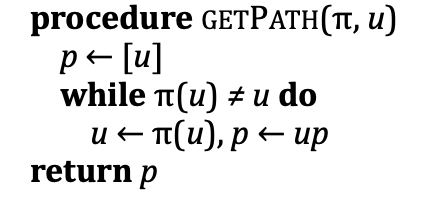## 2. 有权图中的单源最短路径和 Bellman-Ford 算法

$G = \langle V, E\rangle, ~~E = \{(u, v) \vert w(u, v) \neq \infty\}$

$w$ 为图 $G$ 的 权重矩阵, 它存储 图中每条边的权重.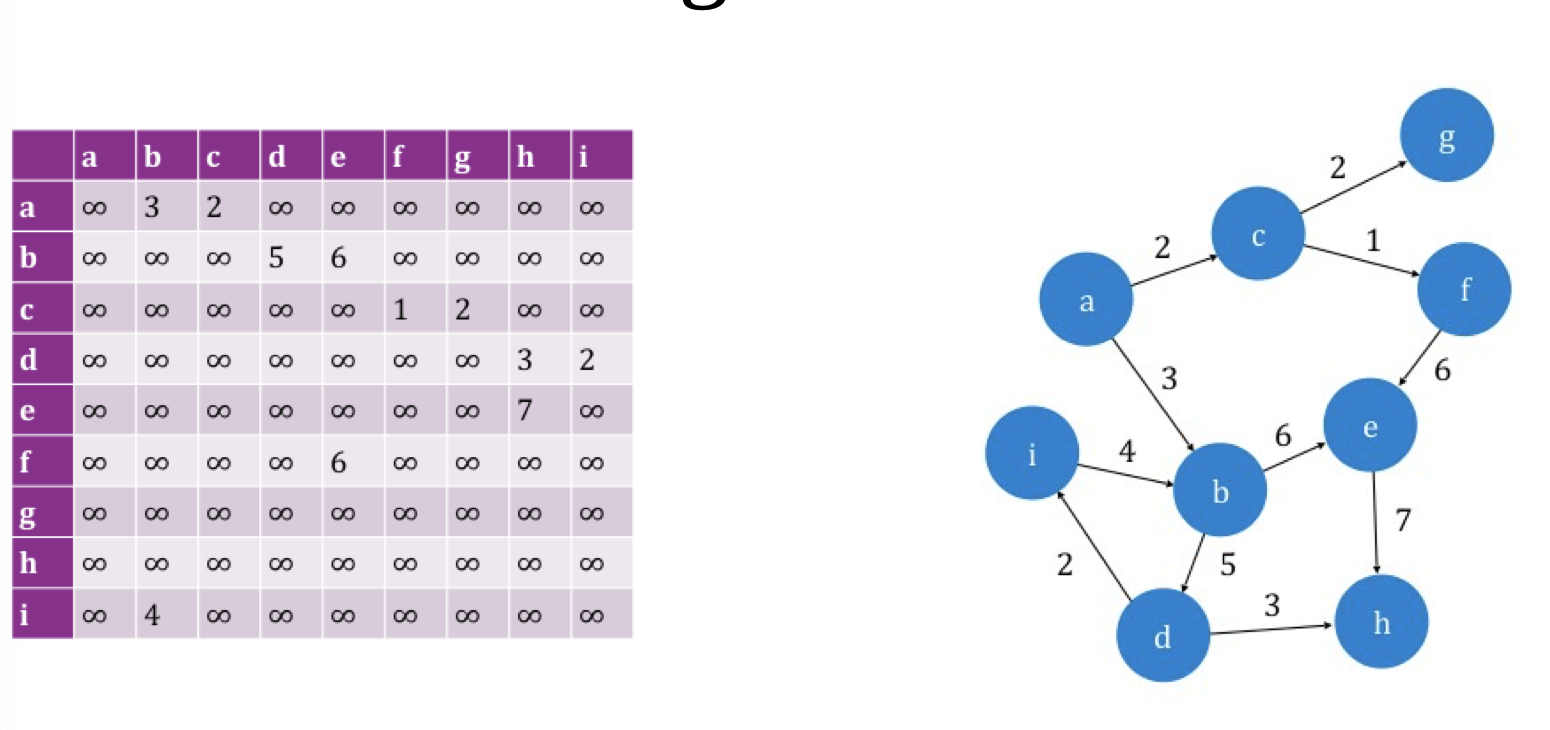1. 我们可以通过从权重矩阵 $w$ 中查值检测节点之间是否连通: 若 $w(u, v) = \infty$, 说明点 $u$ 和 $v$ 之间不存在边.
2. 若 $w(u, v) = d$, 则说明点 $u$ 和 $v$ 之间 存在一条权重为 $d$ 的边.
3. 有权图中的路径和常规的有向图中的路径定义一致, 某条路径的权重即为该路径中全部边的权重之和:

$\vert u_1 \cdots u_n\vert := \sum_{i=1}^{n}w(u_i, u_{i+1})$
4. 对应地, 若某条路径的权重为 $\infty$, 说明这样的路径实际上不存在 (不连通).

$\delta(u, v) := \text{min}\vert u\text{p}v\vert$

$\delta(u, v) = \infty$

Bellman-Ford 算法的基本思路是:

1. 首先对从起始节点到目标节点的路径权重 $\delta(u)$ 的 上界 $D(u)$ 进行估计:

$D(s) = 0, ~~ D(u) = \infty, u \neq s$
2. 循环地 对每条边进行 松弛 (Relax) 操作, 使图的节点集 $V$ 中的每个节点 $v$ 到目标节点 $u$ 的距离估计逐渐接近上确界 (即最短路径长):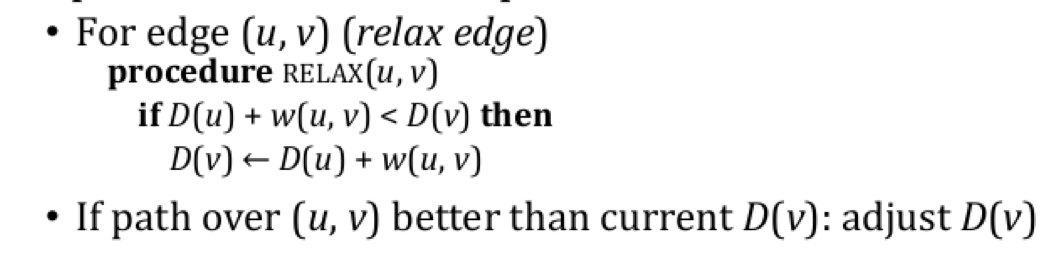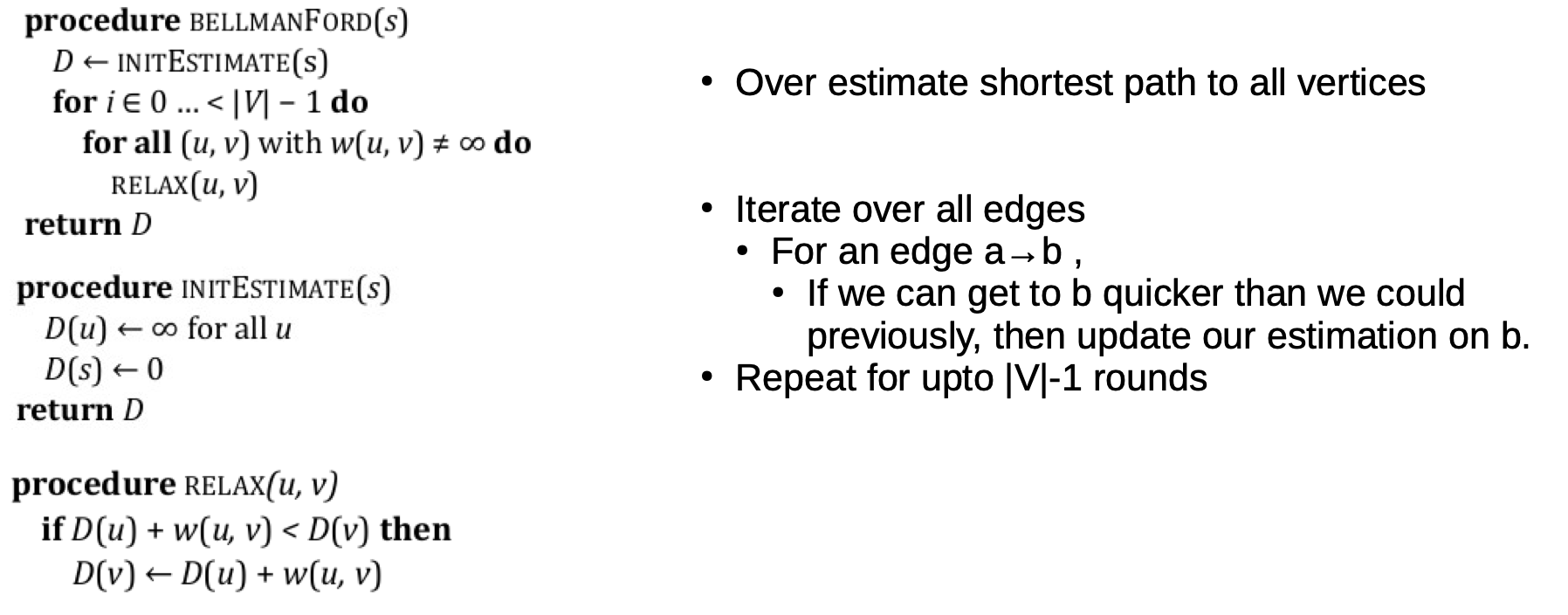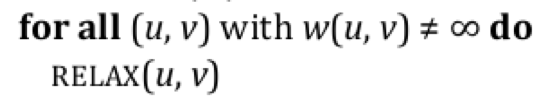## 3. Bellman-Ford 算法的正确性和复杂度分析

### 3.1 算法正确性

1. 在初始循环中就已经成立.
2. 其正确性在任何一次重复的循环中都被保持.
3. 在循环终止后同样保持成立.

1. 从起始节点出发到最远距离为 $I$ 的最短路径 的权重的估计都是精确的.
2. 对任意节点 $u \in V$, 都有 $D(u) \geqslant \delta(u).$

$D(v) = s \rightarrow \mathbf{P} \rightarrow u \rightarrow v$

$D(u) = s \rightarrow \mathbf{P} \rightarrow u$

### 3.3 时间复杂度

$\text{max}(\vert V\vert-1, \vert V \vert) = \vert V\vert$